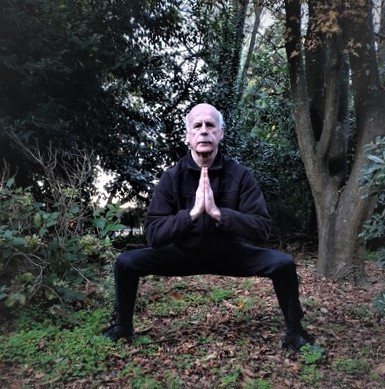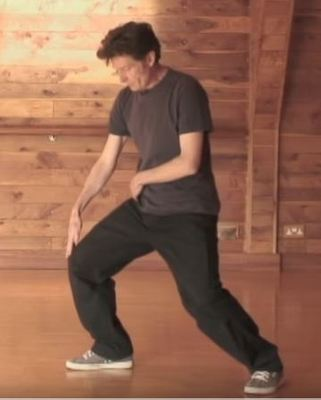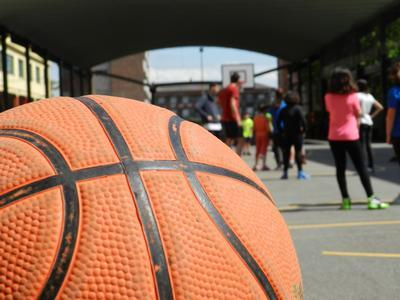Like   Tweet   Pin   +1   in
 /* styles */ Wellbeing + Performance Stories, Tips and Resources   ||   Issue #26 May 2020
 table div table+table+table div table{width:100%;padding:0}table div table+table+table div table img{width:96.23%;padding:0;float:none}table div table+table+table div table td{width:100%;padding:0 1.88% 18px}/* styles */In this issue I share a personal challenge I've set myself, as well as a clip on the importance of the lower tan tien which is essential for good health. You'll also find a Tai Chi tip and a clip on how to protect your knees.

I'm also sharing an interesting article by coach Julia Walsh on what basketball may look like after the pandemic restrictions are eased.

Regards
Chris Bennett
Chris Chi

 table div table+table+table+table+table div table{width:100%;padding:0}table div table+table+table+table+table div table img{width:96.23%;padding:0;float:none}table div table+table+table+table+table div table td{width:100%;padding:0 1.88% 18px}/* styles */## Challenge YourselfChris Bennett

After more than thirty years of Tai Chi practice, I still set myself challenges to improve my skills.

I've avoided the very demanding low stance, because of a concern that it may strain my knees.

I've had a problem with my left knee in the past, but have strengthened that area with Tai Chi training so I can now stay in a high stance and mid level stance for a length of time.

 table div table+table+table+table+table+table+table+table div table{width:100%;padding:0}table div table+table+table+table+table+table+table+table div table img{width:96.23%;padding:0;float:none}table div table+table+table+table+table+table+table+table div table td{width:100%;padding:0 1.88% 18px}/* styles */## Lower Tan TienIn this 3.53 clip Bruce Frantzis describes and emphasises the importance of the lower tan tien for developing Chi.

He says 'if you increase the energy in your lower tan tien you increase your sense of physicality and everything to do with it, whether it's health, power, strength, focus'.

These are some of the areas I cover in all the workshops I run for various groups.

 table div table+table+table+table+table+table+table+table+table+table+table div table{width:100%;padding:0}table div table+table+table+table+table+table+table+table+table+table+table div table img{width:96.23%;padding:0;float:none}table div table+table+table+table+table+table+table+table+table+table+table div table td{width:100%;padding:0 1.88% 18px}/* styles */## Tai Chi Tip

 /* styles */ When performing your Tai Chi movements, breathe in a gentle, smooth, slow and deep manner.
 table div table+table+table+table+table+table+table+table+table+table+table+table+table+table div table{width:100%;padding:0}table div table+table+table+table+table+table+table+table+table+table+table+table+table+table div table img{width:96.23%;padding:0;float:none}table div table+table+table+table+table+table+table+table+table+table+table+table+table+table div table td{width:100%;padding:0 1.88% 18px}/* styles */## How to Protect Your KneesAngus Clarke offers a clear and concise explanation of how to protect your knees from injury. The short clip is only 2.30 minutes long.

In my early days of Tai Chi I practiced incorrectly and strained my knees, so the tips offered in this clip are essential for safe practice.

Now, when I teach, I make sure students are correctly aligned so they can get the full benefits that Tai Chi has to offer.

 table div table+table+table+table+table+table+table+table+table+table+table+table+table+table+table+table+table div table{width:100%;padding:0}table div table+table+table+table+table+table+table+table+table+table+table+table+table+table+table+table+table div table img{width:96.23%;padding:0;float:none}table div table+table+table+table+table+table+table+table+table+table+table+table+table+table+table+table+table div table td{width:100%;padding:0 1.88% 18px}/* styles */## Reimagining Basketball After The PandemicPhoto courtesty of Enrique Rodrigrez Santamaria (via Morgue File)

Here's a very interesting article by sports coach Julia Walsh entitled Reimagining the Game. Part 1 Logistics.

She re-imagines 'what basketball practice and competition might look like' after the corona virus restrictions are eased.

As a former basketball coach, it's a world I could never imagine. But it's everybody's reality now so it's time to adapt and look for the opportunities to move forward.

 table div table+table+table+table+table+table+table+table+table+table+table+table+table+table+table+table+table+table+table+table div table{width:100%;padding:0}table div table+table+table+table+table+table+table+table+table+table+table+table+table+table+table+table+table+table+table+table div table img{width:96.23%;padding:0;float:none}table div table+table+table+table+table+table+table+table+table+table+table+table+table+table+table+table+table+table+table+table div table td{width:100%;padding:0 1.88% 18px}/* styles */table div table+table+table+table+table+table+table+table+table+table+table+table+table+table+table+table+table+table+table+table+table+table div table{width:100%;padding:0}table div table+table+table+table+table+table+table+table+table+table+table+table+table+table+table+table+table+table+table+table+table+table div table img{width:96.23%;padding:0;float:none}table div table+table+table+table+table+table+table+table+table+table+table+table+table+table+table+table+table+table+table+table+table+table div table td{width:100%;padding:0 1.88% 18px}/* styles *//* styles */
 table div table+table+table+table+table+table+table+table+table+table+table+table+table+table+table+table+table+table+table+table+table+table+table+table div table{width:100%;padding:0}table div table+table+table+table+table+table+table+table+table+table+table+table+table+table+table+table+table+table+table+table+table+table+table+table div table img{width:96.23%;padding:0;float:none}table div table+table+table+table+table+table+table+table+table+table+table+table+table+table+table+table+table+table+table+table+table+table+table+table div table td{width:100%;padding:0 1.88% 18px}/* styles *//* styles */